## Hurwitz Polynomial:

For a polynomial P(s) to be a Hurwitz polynomial, it has to satisfy following basic properties,

• The polynomial P(s) is real when ‘s’ is real.
• The roots of the polynomial P(s) have real parts which are either zero or negative.

In addition to these basic properties, Hurwitz polynomial has to satisfy few more properties.

### Properties of Hurwitz Polynomials:

Consider a polynomial P(s) of order ‘n’ as,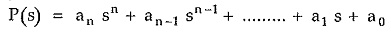where an , an_1 , …  a0 are the coefficients.

For the polynomial P(s) to be Hurwitz,

• All the coefficients ‘ai‘ are real and nonnegative. Thus all the coefficients an to a0 must be positive.
• All the terms starting from highest power of s to lowest power of s must be No power of s should be missing in between.

A corollary to this is that between highest order term in s and lowest order term in s, none of the coefficients may be zero, unless the polynomial is either even or odd.

The polynomial is said to be even if it consists of only even powers of s. In such case, all odd powers of s are missing but in such polynomial also no even power should be missing in between. For example,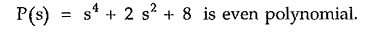Similarly the polynomial is said to be odd if it consists of only odd powers of s. In such case, all even powers of s are missing but in such polynomials also no odd power should be missing in between. For example,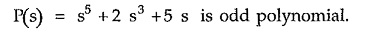• If the polynomial is even or odd then all its roots must lie on imaginary jω axis.
• Given Hurwitz polynomial can be separated into even and odd parts. The odd part is denoted as n(s) while even by m(s) then,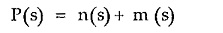Both these n(s) and m(s) parts must have roots on jω axis only.

If the ratio of odd to even parts of P(s) or even to odd parts of P(s) is expressed in the continued fraction expansion then all the quotient terms must be positive.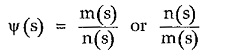Then the continued fraction expansion is,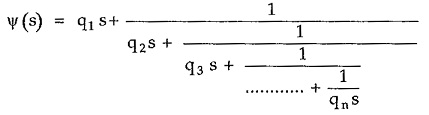All the quotients q1, q…. qn must be positive for the P(s) to be Hurwitz.

The process of obtaining the continued fraction expansion includes simple division and then inversion. At each step we get a quotient qi and a remainder. We then invert a remainder term and then divide to obtain a new quotient. The last remainder must be zero. The continued fraction expansion must be finite in length. To understand this, let us express P(s) in continued fraction expansion.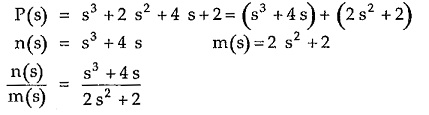Ratio of n(s) to m(s) or m(s) to n (s) is dependent on their powers of s. The ratio of higher power to lesser power is generally taken. Start with the division.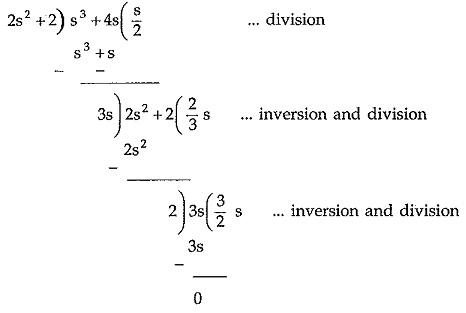In the first step 3s is the remainder. The inversion means 2 s2 +2 is then divided by the remainder 3s. And then division is carried out. In this step, 2 is the remainder. Again 3s is divided by 2 which is an inversion and then division is carried out.

Note that highest power polynomial out of n(s) and m(s) must be considered as a numerator to start the division. Another important observation is that the number of quotients obtained in the continued fraction expansion is equal to highest power of s present in the polynomial P(s).

If the continued fraction expansion gives all the positive quotients then the polynomial P(s) can be expressed as the product of the two factors which are individually Hurwitz. Thus,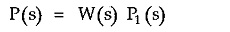then P(s) is Hurwitz if W(s) and P1 (s) are individually Hurwitz.

Continued fraction expansion may terminate abruptly if there exists a common factor between n(s) and m(s) which gets cancelled when the ratio is taken. Then given P(s) can be expressed as the product of the two polynomials as W(s) P1 (s) where W(s) is the common factor present in n(s) and m(s)

Consider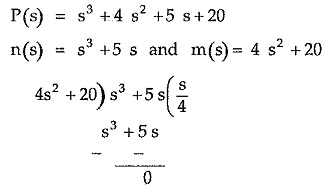The continued fraction terminates abruptly as the number of quotients are not equal to highest power of s in P(s) i.e. 3. Then P(s) can be written as,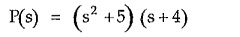where s2 +5 is the common factor which is W(s) and (s + 4) is P1 (s). Both W(s) and P1(s) are Hurwitz and hence P(s) is also Hurwitz.

If P(s) is even or odd then it is not possible to express it in continued fraction form as either n(s) or m(s) is absent. In such case, continued fraction expansion of P(s) with its derivative P'(s) is obtained which has to satisfy all the above properties to have P(s) to be Hurwitz.

The continued fraction expansion of P(s) / P'(s) is obtained.

It can be verified that all the quotients are not positive when P(s) / P'(s) is expressed in continued fraction form, under this case. And hence P(s) is not Hurwitz. The continued fraction expansion is also called Euclid’s algorithm for testing a given polynomial to be Hurwitz or not.

### Routh Hurwitz Array Method:

Another method of testing a given polynomial for Hurwitz is called Routh-Hurwitz array method. In this method, an array is constructed using the coefficients of given polynomial in a specific way. By the inspection of such an array formed, the polynomial can be decided to be Hurwitz or not.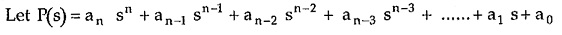The method of finding Routh Hurwitz array is,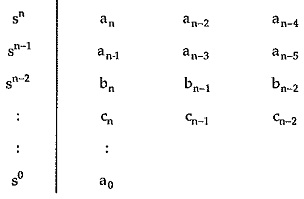In this method, first row consists of all the coefficients of alternate powers of s starting from n while the next row consists of all the coefficients of alternate powers of s starting from n-1. Thus all the coefficients are accommodated in first two rows. Then each next row is generated from the coefficients of the two previous rows to it. So a row corresponding to sn-2 is generated from first two rows as,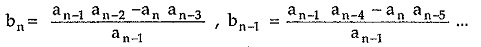The row corresponding to sn-3 is generated from the two previous rows.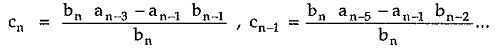The procedure is continued till the row corresponding to s0 is obtained. The last row always consists of a single coefficient which is equal to the constant term of the given polynomial.

Once the array is completed, its first column is inspected. For the given polynomial P(s) to be Hurwitz,

1. All the elements in the first column should be non zero.
2. There should not be any sign change in the first column.

Scroll to Top